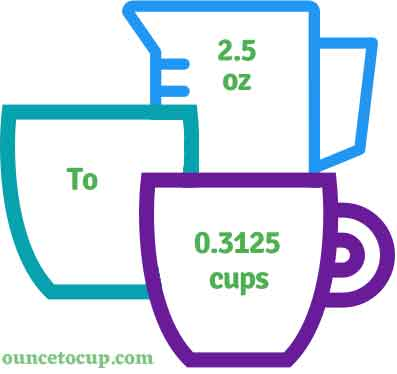# 2.5 Ounce to Cups (2.5 oz to cups conversion)

Are you cooking your favorite dish? The detailed chart in the recipe includes the calculation of 2.5 ounces to cups conversion? Do not worry; check this conversion tool to find how many 2.5 ounces equal to cups in a minute. This 2.5 oz to cup converter gives an exact measurement for any recipe you prepare.

Ounce Value:

Ounces

Cup Value:

Cups

2.5 Ounce = 0.3125 Cup
(2.5 oz = 0.3125 cup)

Try our auto 2.5 ounce to cup calculator (Without Convert Button), Just change the first field value and you got final value.## How many cups is 2.5 oz?

We know that the volume value of 2.5 oz is equal to 0.3125 cups. If you want to convert 2.5 fluid oz to an equal number of cups, just divide the volume value by 8. Hence, 2.5 Ounce is equal to 0.3125 cup.

The Answer is: 2.5 US Fluid Ounces = 0.3125 US Cup

2.5 oz = 0.3125 cup

Many of them try to search or find an answer for what is 2.5 ounces in cups? So, we’ll start with 2.5 fl oz to cups conversion to know how big is 2.5 oz.

## How To Calculate 2.5 fluid oz to cups?

To calculate 2.5 fluid ounces to an equal number of cups, simply follow the steps below.

Fluid Ounces to Cups formula is:

Cup = Fluid Ounce / 8

Assume that we are finding out how many cups were found in 2.5 fl oz of water, multiply it either by 0.125 or divide the value by 8 to get the result.

Applying to Formula: Cup = 2.5 oz / 8 = 0.3125 cup.

## How to convert 2.5 oz to cups?

• To convert 2.5 fluid ounces to cups,
• Simply multiply the 2.5 fluid ounces by 0.125, or simply divide them by 8.
• Applying to the formula, cups = 2.5 ounces * 0.125 [2.5x0.125] (or) cups = 2.5 ounces/8 = 2.5/8 = 0.3125.
• Hence, 2.5 ounces is equal to 0.3125 cups.

## Some quick table references for fluid ounce to cup conversions:

Ounce [oz] Cup [c]
1 oz0.125 cup
2 oz0.25 cup
3 oz0.375 cup
4 oz0.5 cup
5 oz0.625 cup
6 oz0.75 cup
7 oz0.875 cup
8 oz1 cup
9 oz1.125 cup
10 oz1.25 cup
11 oz1.375 cup
12 oz1.5 cup

## Reverse Calculation: How many ounces are in 2.5 cups?

• To convert 2.5 cups to ounces,
• Simply multiply the 2.5 cups by 8.
• Then, applying the formula, ounce = 2.5 cups * 8 [2.5x8 = 20].
• Hence, 2.5 cup is equal to 20 ounces.

### Related Converter:

Formula: Ounce to Cup

Cup = Ounce / 8

Applying to Formula,

Cup = 2.5/8 = 0.3125

2.5 Ounce = 0.3125 Cup